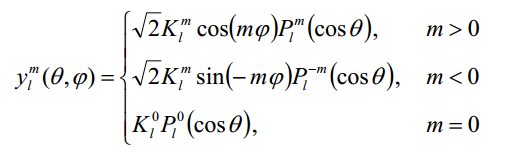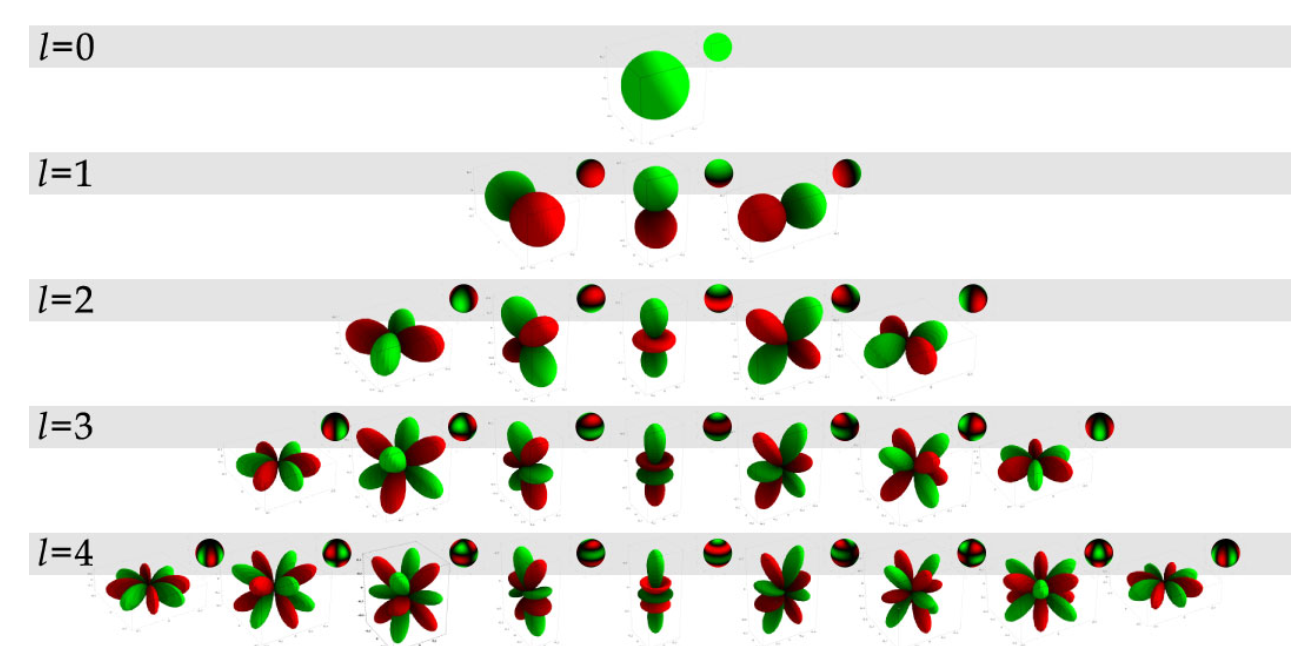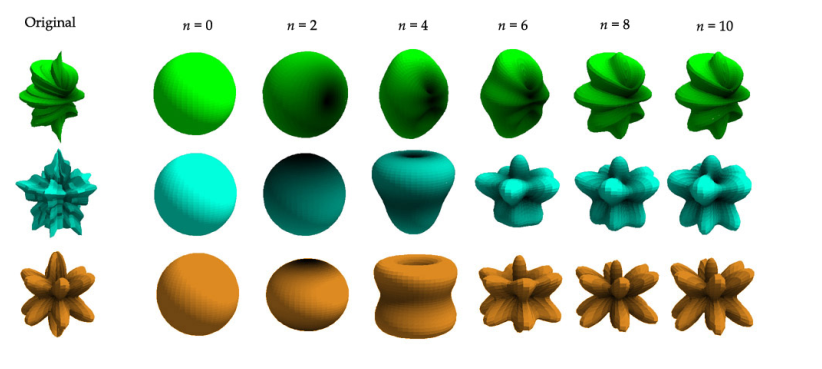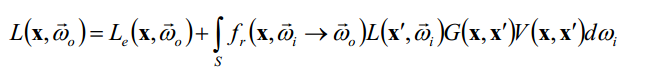# 简单理解spherical harmonic lighting（球谐光照）

## 2.拟合思想和球面调谐spherical harmonic

ok，我们有了正交基底函数的概念，那么就可以拓展到球面调谐函数了。上面的F(X)是在直线x轴上的 ，而渲染中的函数分布一般是基于球面的（下面会提到），球面函数一般可以表述为F(θ，φ）,连个参数是一个规范化球在两个轴的夹角。如何向上面F(X)确定它的正交基底呢。球面函数的也有例如傅里叶展开一样的东西，叫做勒让德多项式（Legendre Polynomials），勒让德多项式里的基地函数分为几组，每组有一个index，其中index越高，频率越高，每个组不是单独的一个基底，第n组有（n+1）²  - 1个基地函数组成，用y（l,m）代表第l组里面的第m个基底，所有的基底都是正交的，利用勒让德多项式可以得到球面函数的基底的定义是band 0里各个位置是一样的，可以认为是个平均值，而后面的band越高，越表示了高频信息。## 3.球谐光照## 4.Unity中的球谐光照应用

unity中至少在光照探头和前向渲染的大量顶点光照这两个地方上使用了球谐光照的技术。

unity的光照探头在烘焙的时候为每个探头点附近采样光照值，然后计算每个点的球谐函数基底系数，用于运行时对于动态物体计算当前点的烘焙时的全局光照。

inline half4 VertexGIForward(VertexInput v, float3 posWorld, half3 normalWorld)
{
half4 ambientOrLightmapUV = 0;
// Static lightmaps
#ifdef LIGHTMAP_ON
ambientOrLightmapUV.xy = v.uv1.xy * unity_LightmapST.xy + unity_LightmapST.zw;
ambientOrLightmapUV.zw = 0;
// Sample light probe for Dynamic objects only (no static or dynamic lightmaps)
#elif UNITY_SHOULD_SAMPLE_SH
#ifdef VERTEXLIGHT_ON
// Approximated illumination from non-important point lights
ambientOrLightmapUV.rgb = Shade4PointLights (
unity_4LightPosX0, unity_4LightPosY0, unity_4LightPosZ0,
unity_LightColor.rgb, unity_LightColor.rgb, unity_LightColor.rgb, unity_LightColor.rgb,
unity_4LightAtten0, posWorld, normalWorld);
#endif

ambientOrLightmapUV.rgb = ShadeSHPerVertex (normalWorld, ambientOrLightmapUV.rgb);
#endif

#ifdef DYNAMICLIGHTMAP_ON
ambientOrLightmapUV.zw = v.uv2.xy * unity_DynamicLightmapST.xy + unity_DynamicLightmapST.zw;
#endif

return ambientOrLightmapUV;
}

half3 ShadeSHPerVertex (half3 normal, half3 ambient)
{
#if UNITY_SAMPLE_FULL_SH_PER_PIXEL
// Completely per-pixel
// nothing to do here
#elif (SHADER_TARGET < 30) || UNITY_STANDARD_SIMPLE
// Completely per-vertex
ambient += max(half3(0,0,0), ShadeSH9 (half4(normal, 1.0)));
#else
// L2 per-vertex, L0..L1 & gamma-correction per-pixel

// NOTE: SH data is always in Linear AND calculation is split between vertex & pixel
// Convert ambient to Linear and do final gamma-correction at the end (per-pixel)
#ifdef UNITY_COLORSPACE_GAMMA
ambient = GammaToLinearSpace (ambient);
#endif
ambient += SHEvalLinearL2 (half4(normal, 1.0));     // no max since this is only L2 contribution
#endif

return ambient;
}

// normal should be normalized, w=1.0
half3 SHEvalLinearL0L1 (half4 normal)
{
half3 x;

// Linear (L1) + constant (L0) polynomial terms
x.r = dot(unity_SHAr,normal);
x.g = dot(unity_SHAg,normal);
x.b = dot(unity_SHAb,normal);

return x;
}

// normal should be normalized, w=1.0
half3 SHEvalLinearL2 (half4 normal)
{
half3 x1, x2;
// 4 of the quadratic (L2) polynomials
half4 vB = normal.xyzz * normal.yzzx;
x1.r = dot(unity_SHBr,vB);
x1.g = dot(unity_SHBg,vB);
x1.b = dot(unity_SHBb,vB);

// Final (5th) quadratic (L2) polynomial
half vC = normal.x*normal.x - normal.y*normal.y;
x2 = unity_SHC.rgb * vC;

return x1 + x2;
}

// normal should be normalized, w=1.0
// output in active color space
half3 ShadeSH9 (half4 normal)
{
// Linear + constant polynomial terms
half3 res = SHEvalLinearL0L1 (normal);

res += SHEvalLinearL2 (normal);

#   ifdef UNITY_COLORSPACE_GAMMA
res = LinearToGammaSpace (res);
#   endif

return res;
}

# 参考论文

http://silviojemma.com/public/papers/lighting/spherical-harmonic-lighting.pdf